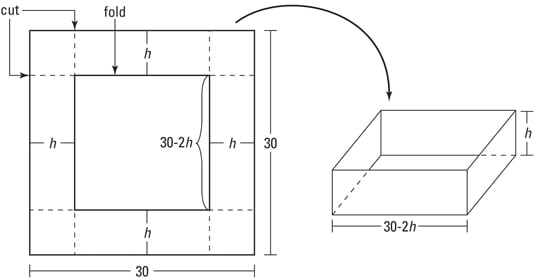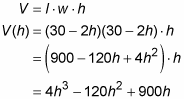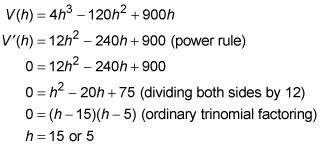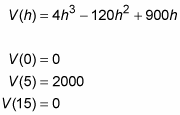##### Trigonometry Workbook For DummiesOne of the most practical uses of differentiation is finding the maximum or minimum value of a real-world function. In the following example, you calculate the maximum volume of a box that has no top and that is to be manufactured from a 30-inch-by-30-inch piece of cardboard by cutting and folding it as shown in the figure.What dimensions produce a box that has the maximum volume? Mathematics often seems abstract and impractical, but here’s an honest-to-goodness practical problem. If a manufacturer can sell bigger boxes for more money, and he or she is making a million boxes, you better believe he or she will want the exact answer to this question:

1. Express the thing you want maximized, the volume, as a function of the unknown, the height of the box (which is the same as the length of the cut).2. Determine the domain of your function.

The height can’t be negative or greater than 15 inches (the cardboard is only 30 inches wide, so half of that is the maximum height). Thus, sensible values for h are 0 ≤ h ≤ 15.

3. Find the critical numbers of V(h) in the open interval (0, 15) by setting its derivative equal to zero and solving. And don’t forget to check for numbers where the derivative is undefined.Because 15 isn't in the open interval (0, 15), it doesn’t qualify as a critical number. And because this derivative is defined for all input values, there are no additional critical numbers. So, 5 is the only critical number.

4. Evaluate the function at the critical number, 5, and at the endpoints of the interval, 0 and 15, to locate the function’s max.The extremum (dig that fancy word for maximum or minimum) you’re looking for doesn’t often occur at an endpoint, but it can — so don’t fail to evaluate the function at the interval’s two endpoints.

You’ve got your answer: a height of 5 inches produces the box with maximum volume (2000 cubic inches). Because the length and width equal 30 – 2h, a height of 5 inches gives a length and width of 30 – 2 · 5, or 20 inches. Thus, the dimensions of the desired box are 5 inches by 20 inches by 20 inches.